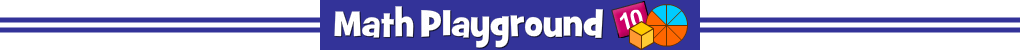Meteor Multiplication
The multiplication meteors are coming closer. Can you save the day?
Practice multiplication facts and keep our planet safe!
Meteor Multiplication - Learning Connections
Essential Skills
Mental Math - multiply numbers by memory or through other efficient methods
Problem Solving - match multiplication expressions with the correct products

Common Core Connection for 3rd Grade
Determine the unknown whole number in a multiplication equation (4 x 6 = ?).
Apply properties of operations as strategies to multiply.